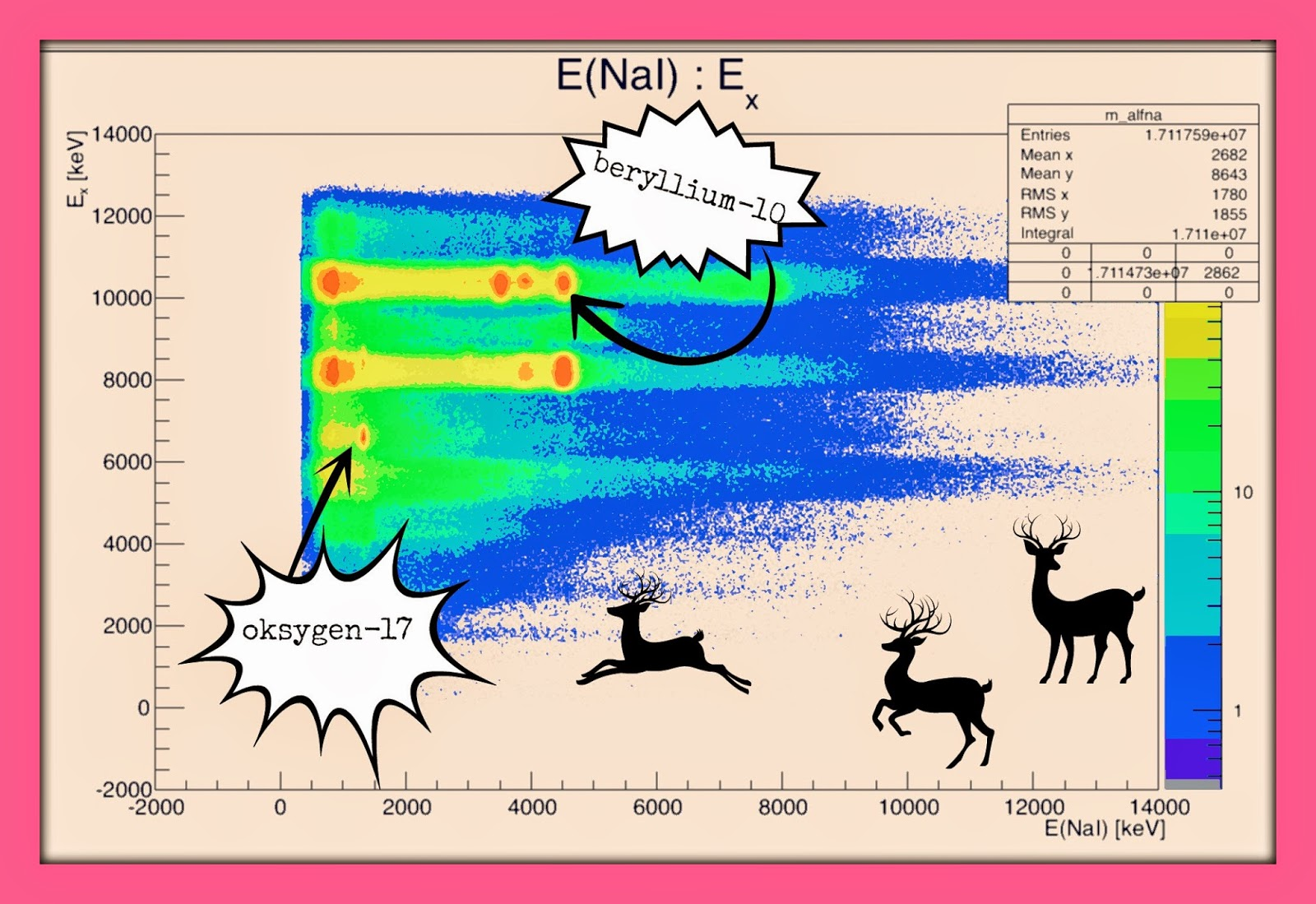torsdag 18. desember 2014

Number 18

So, in the calendar today there are gammas (gamma radiation) - I´m giving you an ALFNA matrix :D xD <3The ALFNA matrix/plot is a sort of 3 dimensional plot where there is the excitation energy of some nucleus (we don´t know which one - and this is what we/I try to figure out) on the y-axis, and on the x-axis is the energy of the gamma(s) the nucleus sends out. The thing is that when a nucleus is hit by a particle, the nucleus is excited, or sort of heated - it gets some extra energy, and to get rid of that extra energy it sends out  gamma radiation.
From earlier experiments that have been done (the bible is here) we know quite a lot about what kind of gammas (which energies) the different nuclei will emit when they are excited to this and that energy. In this particular ALFNA matrix we believe that we have oxygen-17 (there is always some oxygen :) ) and beryllium-10 (the backing of the uranium foil/target is made from beryllium). The oxygen-17 peak for example, is supposed to emit a gamma ray with an energy of 870 kilo electron volts (keV), and here it is at around 1300 keV - which means that we are quite off, and everything needs to be calibrated (which is exactly what I´m working on now ;) ).

So this is the plot I´ve been staring at today (together with my supervisor, Sunniva <3).
...and I´m staring even more now (pretending to have a really huge brain) - looking like this :P